# Machine Learning using Python Interview Questions Data Science

Prepare better with the best interview questions and answers, and walk away with top interview tips. These interview questions and answers will boost your core interview skills and help you perform better. Be smarter with every interview.

• 4.5 Rating
• 25 Question(s)

## Beginner

• K-Nearest Neighbors is a supervised classification algorithm, while k-means clustering is an unsupervised clustering algorithm. While the mechanisms may seem similar at first, what this really means is that in order for K-Nearest Neighbors to work, you need labelled data you want to classify an unlabeled point into (thus the nearest neighbour part)
• K-Nearest Neighbour is a simple algorithm that stores all available cases and predicts the target based on a similarity measure.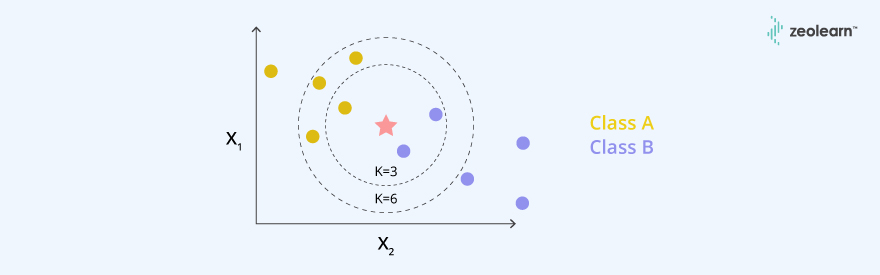Fig1. KNN

Example: K-Nearest Neighbors (K-NN)

```# Importing the libraries
import numpy as np
import matplotlib.pyplot as plt
import pandas as pd

# Importing the dataset
X = dataset.iloc[:, [2, 3]].values
y = dataset.iloc[:, 4].values

# Splitting the dataset into the Training set and Test set
from sklearn.cross_validation import train_test_split
X_train, X_test, y_train, y_test = train_test_split(X, y, test_size = 0.25, random_state = 0)

# Feature Scaling
from sklearn.preprocessing import StandardScaler
sc = StandardScaler()
X_train = sc.fit_transform(X_train)
X_test = sc.transform(X_test)

# Fitting K-NN to the Training set
from sklearn.neighbors import KNeighborsClassifier
classifier = KNeighborsClassifier(n_neighbors = 5, metric = 'minkowski', p = 2)
classifier.fit(X_train, y_train)

# Predicting the Test set results
y_pred = classifier.predict(X_test)```

K-means clustering requires only a set of unlabeled points and a threshold: the algorithm will take unlabeled points and gradually learn how to cluster them into groups by computing the centroid of the distance between different points.

In total there are 4 related decisions that need to be taken for the approach :

1. Initialize the set of means (centroid of clusters you want to find)
2. Assign the factor the nearest mean
3. Once, this is done we compute the centroids of the clusters that are found and make them the new means
4. Repeat steps 2,3 until they don’t change anymore.

Sooner or later k-Means converge when the clusters no longer will change. (In our case we stop after a number of iterations)

The critical difference here is that KNN needs labelled points and is thus supervised learning, while k-means doesn’t and is thus unsupervised learning.

# Example: K-means

```# Importing the libraries
import numpy as np
import matplotlib.pyplot as plt
import pandas as pd

# Importing the dataset
X = dataset.iloc[:, [3, 4]].values

# Using the elbow method to find the optimal number of clusters
from sklearn.cluster import KMeans
wcss = []
for i in range(1, 11):
kmeans = KMeans(n_clusters = i, init = 'k-means++', random_state = 42)
kmeans.fit(X)
wcss.append(kmeans.inertia_)
plt.plot(range(1, 11), wcss)
plt.title('The Elbow Method')
plt.xlabel('Number of clusters')
plt.ylabel('WCSS')
plt.show()

# Fitting K-Means to the dataset
kmeans = KMeans(n_clusters = 5, init = 'k-means++', random_state = 42)
y_kmeans = kmeans.fit_predict(X)```

Pruning is what happens in decision trees when branches that have weak predictive power are removed in order to reduce the complexity of the model and increase the predictive accuracy of a decision tree model. You prune it by replacing each node and keep pruning unless predictive accuracy is decreased.

#Example:

If the training set accuracy is 100%, then we are likely to be overfitting. To reduce this overfitting, we could either apply stronger pre-pruning by limiting the maximum depth or tune the learning rate.

# Example:

```from sklearn.tree import DecisionTreeClassifier
tree = DecisionTreeClassifier (max_depth=4, random_state=0)
tree.fit(X_train, y_train)```

Model accuracy is a subset of model performance.  So there’s no right answer to it. For example, if you wanted to detect fraud in a massive dataset with a sample of millions, a more accurate model would most likely predict no fraud at all if only a vast minority of cases were fraud. However, this would be useless for a predictive model — a model designed to find fraud that asserted there was no fraud at all.

#Example: Below is an example of calculating classification accuracy.

```# Cross Validation Classification Accuracy
import pandas as pd
from sklearn import model_selection
from sklearn.linear_model import LogisticRegression
array = dataframe.values
X = Predictors
Y = Response variable
kfold = model_selection.KFold(n_splits=10, random_state=4)
model = LogisticRegression()
scoring = 'accuracy'
results = model_selection.cross_val_score(model, X, Y, cv=kfold, scoring=scoring)
print("Accuracy: %.3f (%.3f)") % (results.mean(), results.std())```

A regression model that uses L1 regularization technique is called Lasso Regression and model which uses L2 is called Ridge Regression. The key difference between these two is the penalty term.

Ridge regression adds “squared magnitude” of coefficient as penalty term to the loss function. Here the highlighted part represents L2 regularization element.Here, if lambda is zero then you can imagine we get back OLS. However, if lambda is very large then it will add too much weight and it will lead to under-fitting. Having said that it’s important how lambda is chosen. This technique works very well to avoid over-fitting issue.

Lasso Regression (Least Absolute Shrinkage and Selection Operator) adds “absolute value of magnitude” of coefficient as penalty term to the loss function.Again, if lambda is zero then we will get back OLS whereas very large value will make coefficients zero hence it will under-fit.

#Example: Below is an implementation for ‘L1’ regularization paramter

```from sklearn.svm import LinearSVC
from sklearn.feature_selection import SelectFromModel
X, y = iris.data, iris.target

lsvc = LinearSVC(C=0.01, penalty="l1", dual=False).fit(X, y)
model = SelectFromModel(lsvc, prefit=True)
X_new = model.transform(X)```
• The training set is a subset of your data on which your model will learn how to predict the dependent variable with the independent variables. The test set is the complementary subset from the training set, on which you will evaluate your model to see if it manages to predict correctly the dependent variable with the independent variables.
•  We split on the dependent variable because we want to have well-distributed values of the dependent variable in the training and test set. For example, if we only had the same value of the dependent variable in the training set, our model wouldn’t be able to learn any correlation between the independent and dependent variables.
```import numpy as np
from sklearn.model_selection import train_test_split
from sklearn import datasets
from sklearn import svm

X_train, X_test, y_train, y_test = train_test_split( iris.data, iris.target, test_size=0.4, random_state=0)

clf = svm.SVC(kernel='linear', C=1).fit(X_train, y_train)
clf.score(X_test, y_test) ```

Recall is also known as the true positive rate: the number of positives your model claims compared to the actual number of positives there are throughout the data.

Recall: TP / (TP + FN)

Precision is also known as the positive predictive value, and it is a measure of the number of accurate positives your model claims compared to the number of positives it actually claims.

Precision: TP / (TP + FP)

#Example:

Suppose you’ve predicted that there were 10 apples and 5 oranges in a case of 10 apples. You’d have perfect recall (there are actually 10 apples, and you predicted there would be 10) but 66.7% precision because out of the 15 events you predicted, only 10 (the apples) are correct.

```from sklearn import metrics

‘Precision Score': metrics.precision_score(y_test, y_pred)
'Recall Score':    metrics.recall_score(y_test, y_pred)```
• Collect more data to even the imbalances in the dataset,
• Resample the dataset to correct for imbalances (Undersampling/ Oversampling),
• Try a different algorithm altogether on your dataset (Bagging or boosting classifiers),
• Generate synthetic samples (S.M.O.T.E)

a) In the meshgrid() method, you input two arguments:

The first argument is the range values of the x-coordinates in your grid.

Second is the range values of the y-coordinates in your grid.

So let’s say that these 1st and 2nd arguments are respectively [-1,+1] and [0,10], then you will get a grid where the values will go from [-1,+1] on the x-axis and [0,10] on the y-axis.

b) Before using the contourf method, you need to build a meshgrid. Then, the contourf() method takes several arguments such as:

1.  The range values of the x-coordinates of your grid,
2.  The range values of the y-coordinates of your grid,
3.  A fitting line (or curve) that will be plotted in this grid (we plot this fitting line using the predict function because this line are the continuous predictions of our model),
4.  Then the rest are optional arguments like the colours to plot regions of different colours.

The regions will be separated by this fitting line, that is, in fact, the contour line.

#Example –  Below is an implementation of the following visual methods:

```# Logistic Regression

# Importing the libraries
import numpy as np
import matplotlib.pyplot as plt
import pandas as pd

# Importing the dataset
X = dataset.iloc[:, [2, 3]].values
y = dataset.iloc[:, 4].values

# Splitting the dataset into the Training set and Test set
from sklearn.cross_validation import train_test_split
X_train, X_test, y_train, y_test = train_test_split(X, y, test_size = 0.25, random_state = 0)

# Feature Scaling
from sklearn.preprocessing import StandardScaler
sc = StandardScaler()
X_train = sc.fit_transform(X_train)
X_test = sc.transform(X_test)

# Fitting Logistic Regression to the Training set
from sklearn.linear_model import LogisticRegression
classifier = LogisticRegression(random_state = 0)
classifier.fit(X_train, y_train)

# Predicting the Test set results
y_pred = classifier.predict(X_test)

# Visualising the Training set results
from matplotlib.colors import ListedColormap
X_set, y_set = X_train, y_train
X1, X2 = np.meshgrid(np.arange(start = X_set[:, 0].min() - 1, stop = X_set[:, 0].max() + 1, step = 0.01),np.arange(start = X_set[:, 1].min() - 1, stop = X_set[:, 1].max() + 1, step = 0.01))

plt.contourf(X1, X2, classifier.predict(np.array([X1.ravel(), X2.ravel()]).T).reshape(X1.shape),
alpha = 0.75, cmap = ListedColormap(('red', 'green')))
plt.xlim(X1.min(), X1.max())
plt.ylim(X2.min(), X2.max())

for i, j in enumerate(np.unique(y_set)):
plt.scatter(X_set[y_set == j, 0], X_set[y_set == j, 1],
c = ListedColormap(('red', 'green'))(i), label = j)
plt.title('Logistic Regression (Training set)')
plt.xlabel('Age')
plt.ylabel('Estimated Salary')
plt.legend()
plt.show()```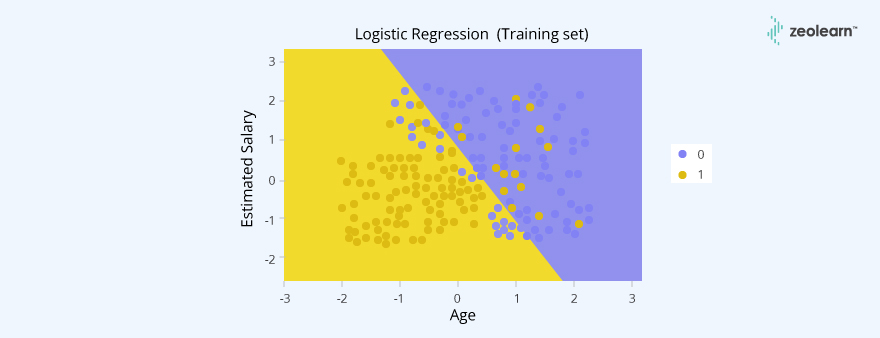SVM uses hinge loss function:Where w^2 is the regularize and is the loss function.• Mean removal - It involves removing the mean from each feature so that it is centred on zero. Mean removal helps in removing any bias from the features.
• Feature scaling - The values of every feature in a data point can vary between random values. So, it is important to scale them so that this matches specified rules.
• Normalization - Normalization involves adjusting the values in the feature vector so as to measure them on a common scale. Here, the values of a feature vector are adjusted so that they sum up to 1.
• Binarization - Binarization is used to convert a numerical feature vector into a Boolean vector.

#Example:

Standardization

```import numpy as np
from sklearn import preprocessing
X_scaled = preprocessing.MinmaxScaler(feature_range = (0,1))
Data = X_scaled.fit_transform(df) (where df is the Input data.) ```

Normalization

```import numpy as np
from sklearn import preprocessing
X_normalized = preprocessing.normalize(X, norm = ‘l2’)```

We can visualize the data using 2 types of plots :

1. Univariate plots for each individual variable such as Box plot, histogram
2. Multivariate plots such as Scatterplot matrix to understand structured relationship/interactions b/w the variables.

#Example

-Univariate Plots

```import pandas
import matplotlib.pyplot as plt
data = 'iris_df.csv'
names = ['sepal-length', 'sepal-width', 'petal-length', 'petal-width', 'class']
dataset.plot(kind='box', subplots=True, layout=(2,2), sharex=False, sharey=False)
plt.show()```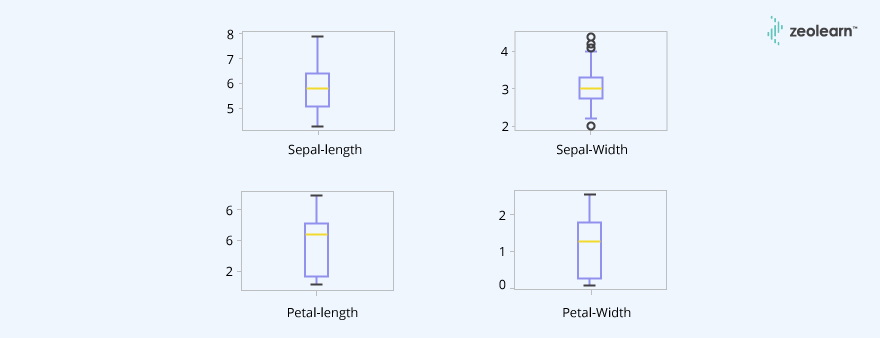-Multivariate Plots

```from pandas.plotting import scatter_matrix

scatter_matrix(dataset)
plt.show()```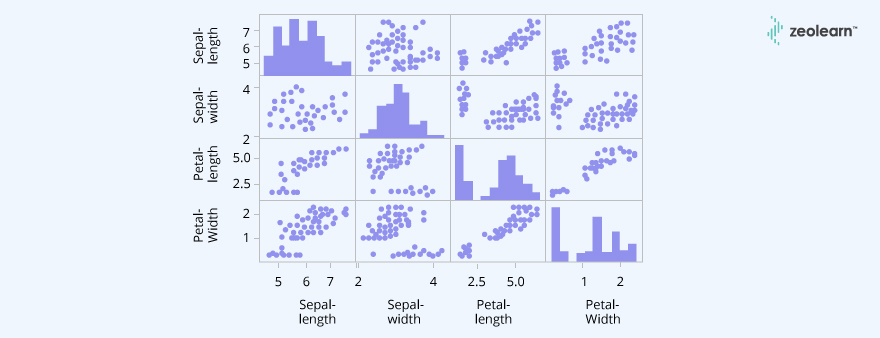There are 4 key hyperparams required for RF:

1. N_estimators – Number of trees in the forest
2. Max_features – No of features to consider at each split. By default: It takes the square root of the total number of features.
3. Max_depth – Max_depth of the trees represent the number of nodes
4. Min_samples_leaf – Min number of samples required to be at a leaf node/ bottom of a tree (min_samples_leaf)

# Example:

```import pandas as pd
from sklearn.model_selection import KFold
from sklearn.model_selection import cross_val_score
from sklearn.ensemble import RandomForestClassifier
array = dataframe.values
X = Predictors
Y = Response variable
n_trees = 100
max_features = 3
kfold = KFold(n_splits=10, random_state=4)
model = RandomForestClassifier(n_estimators = n_trees, max_features = max_features)
results = cross_val_score(model, X, Y, cv=kfold)```

It can be implemented this way:

```import statsmodels.formula.api as sm
def backwardElimination(x, sl):
numVars = len(x)
for i in range(0, numVars):
regressor_OLS = sm.OLS(y, x).fit()
maxVar = max(regressor_OLS.pvalues).astype(float)
if maxVar > sl:
for j in range(0, numVars - i):
if (regressor_OLS.pvalues[j].astype(float) == maxVar):
x = np.delete(x, j, 1)
regressor_OLS.summary()
return x
SL = 0.05
X_opt =  X[:, [0, 1, 2, 3, 4, 5]]
X_Modeled = backwardElimination(X_opt, SL)```
•  The term ‘Boosting’ refers to a family of algorithms which converts weak learner to strong learners. It is a sequential process, where each subsequent model attempts to correct the errors     of  the previous model.

It is an ensemble algorithm that is focused on reducing bias, makes the boosting algorithms prone to overfitting.

To avoid overfitting, parameter tuning plays an important role in boosting algorithms. Some examples of boosting are XGBoost, GBM, ADABOOST, etc.

•  To find weak learners, we apply base learning (ML) algorithms with a different distribution. Each time base learning algorithm is applied, it generates a new weak prediction rule. This is an   iterative process

After many iterations, the boosting algorithm combines these weak rules into a single strong prediction rule

#Example:

Below is an implementation of ADABOOST Classifier with 100 trees and learning rate equals 1

```#Importing necessary packages/Lib
import pandas as pd
import numpy as np
from sklearn.model_selection import train_test_split

#create feature & response variables
X = df_breastcancer.iloc[:,2:31]  # drop the response var and id column as it'll not make any sense to the analysis
Y = df_breastcancer.iloc[:,0] #Target

# Create train & test sets
X_train, X_test, Y_train, Y_test = train_test_split(X, Y, test_size=0.30, random_state=1, stratify= Y)

• The Information Gain in Decision Tree Regression is exactly the Standard Deviation Reduction we are looking to reach. We calculate by how much the Standard Deviation decreases after each split. Because the more the Standard Deviation is decreased after a split, the more homogeneous the child nodes will be.
•  The Entropy measures the disorder in a set, here in a part resulting from a split. So the more homogeneous is your data in a part, the lower will be the entropy. The more you have split, the more you have the chance to find parts in which your data is homogeneous, and therefore the lower will be the entropy (close to 0) in these parts. However you might still find some nodes where the data is not homogeneous, and therefore the entropy would not be that small.
• #Example:
```from sklearn import tree
X = [[0, 0], [2, 2]]
y = [0.5, 2.5]
clf = tree.DecisionTreeRegressor()
clf = clf.fit(X, y)
clf.predict([[1, 1]])```

Differencing can help stabilize the mean of a time series by removing changes in the level of a time series, and so eliminating trend and seasonality.

#Example:

`differenced_data = timeseriesdata. diff( ) `

Gini coefficient, also known as the normalized Gini Index is nothing but the ratio between area between the ROC curve and the diagonal line & the area of the above triangle. It is a measure of statistical dispersion which is sometimes used in classification problems & can be straight away derived from the AUC ROC number.

`GINI = 2* AUC – 1, where Gini above 60% is a ‘good model’ `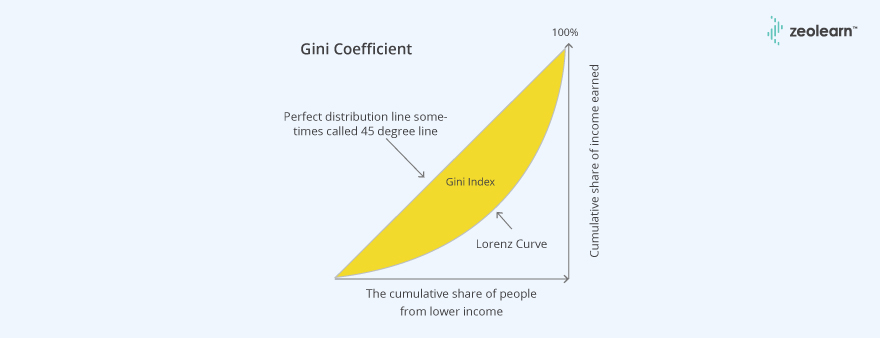PCA is a dimensionality reduction algorithm: PCA takes the data and decomposes it using transformations into principal components (PC). It is a statistical procedure that uses an orthogonal transformation to convert a set of observations of possibly correlated variables (entities each of which takes on various numerical values) into a set of values of linearly uncorrelated variables called principal components.

# Example: Below is an implementation of PCA

```import numpy as np
import pandas as pd

# Breast cancer dataset
(X_cancer, y_cancer) = load_breast_cancer(return_X_y = True)

# Dimensionality Reduction and Manifold Learning
# Principal Components Analysis (PCA)
# Using PCA to find the first two principal components of the breast cancer dataset

from sklearn.preprocessing import StandardScaler
from sklearn.decomposition import PCA

(X_cancer, y_cancer) = load_breast_cancer(return_X_y = True)

# Before applying PCA, each feature should be centered (zero mean) and with unit variance
X_normalized = StandardScaler().fit(X_cancer).transform(X_cancer)

pca = PCA(n_components = 2).fit(X_normalized)

X_pca = pca.transform(X_normalized)
print(X_cancer.shape, X_pca.shape)```

It is a decompositional approach that uses perceptual mapping to present the dimensions. The purpose of the MDS is to transform consumer judgements into distances represented in the multi-dimensional space.

As an exploratory technique, it is useful in examining the unrecognized dimensions about the products and uncovering the comparative evaluation of the products when the basis of comparison is unknown.

# Example: Below is an implementation of MDS on the breast cancer dataset.

```from sklearn.preprocessing import StandardScaler
from sklearn.manifold import MDS

(X_cancer, y_cancer) = load_breast_cancer(return_X_y = True)
# each feature should be centered (zero mean) and with unit variance
X_normalized = StandardScaler().fit(X_cancer).transform(X_cancer)

mds = MDS(n_components = 2)

X_mds = mds.fit_transform(X_normalized)```

One of the important assumptions of linear regression is that there should be no heteroscedasticity of residuals. In simpler terms, this means that the variance of residuals should not increase with fitted values of the response variable.

The reason being, we check if the model thus built is unable to explain some pattern in the response variable that eventually shows up in the residuals. This would result in an inefficient and unstable regression model that could yield bizarre predictions later on. i.e. having falsified/inflated standard error will also disturb the T-value, and as a result, can lead us to accept the P-value which may not be the case sometime.

# Example: Below is the implementation of Breusch pragan test to detect the heteroscedasticity in the linear regression model, where Null hypothesis states that there is no heteroscedasticity.

```from statsmodels.compat import lzip
import statsmodels
import numpy as np
import pandas as pd
import statsmodels.formula.api as smf
import statsmodels.stats.api as sms

results = smf.ols('Response_var ~ Predictors’, data = data).fit()

# Implenting Breusch pragan Test
name = ['Lagrange multiplier statistic', 'p-value', 'f-value', 'f p-value']
test = sms.het_breushpagan(results.resid, results.model.exog)
lzip(name, test)```

# Where Lagrange multiplier is -

• It helps to find an optimal point for a constrained optimization problem
• It can deal with both equality and inequality constraints
• It helps to convert an optimization problem into a system of equations.
• It is the rate of change of the objective function with respect to constant (c) in the constraint (right-hand side of a constraint) at any c equals the Lagrange multiplier at that point.Kernel is a way of computing the dot product of two vectors xx and yy in some (possibly very high dimensional) feature space, which is why kernel functions are sometimes called "generalized dot product".

The Kernel trick involves kernel functions that can enable in higher-dimension spaces without explicitly calculating the coordinates of points within that dimension: instead, kernel functions compute the inner products between the images of all pairs of data in a feature space. This allows them the very useful attribute of calculating the coordinates of higher dimensions while being computationally cheaper than the explicit calculation of said coordinates. Many algorithms can be expressed in terms of inner products. Using the kernel trick enables us to effectively run algorithms in a high-dimensional space with lower-dimensional data.

# Example: SVM has a technique called the kernel trick. These are functions which take low dimensional input space and transform it to a higher dimensional space i.e. it converts not separable problem to separable problem, these functions are called kernels. It is mostly useful in non-linear separation problem. Simply put, it does some extremely complex data transformations, then find out the process to separate the data based on the labels or outputs you’ve defined.

```having linear kernel
import numpy as np
import matplotlib.pyplot as plt
from sklearn import svm, datasets
# import data
X = iris.data[:, :2] # we only take the first two features.
y = iris.target
C = 1.0 # SVM regularization parameter
svc = svm.SVC(kernel='linear', C=1).fit(X, y)```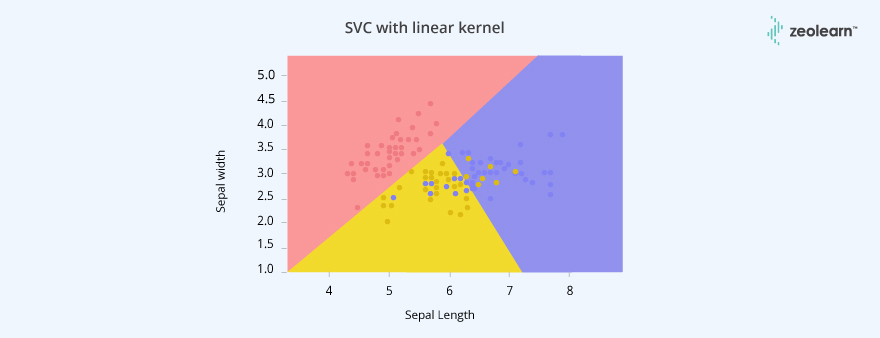```having rbf kernel
svc_2 = svm.SVC(kernel='rbf', C=1).fit(X, y)```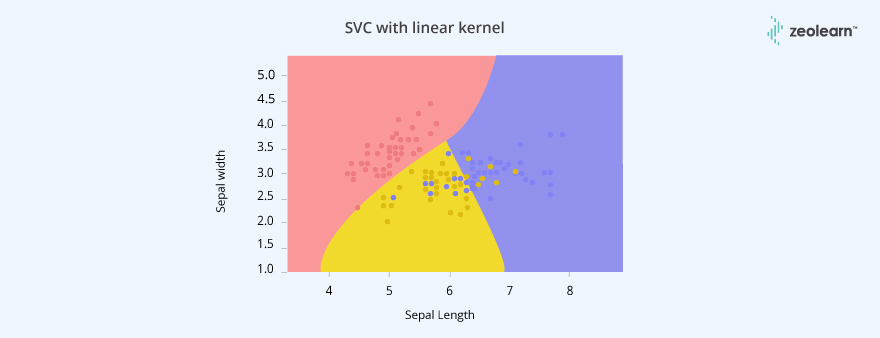Where, gamma: Kernel coefficient for ‘rbf’, ‘poly’ and ‘sigmoid’.

Higher the value of gamma, will try to exactly fit, as per training data set i.e. generalization error and cause over-fitting problem.

& being the penalty parameter of the error term. It also controls the tradeoff between smooth decision boundary and classifying the training points correctly.

Assuming that we don’t know the population mean for the sample. So, we need to calculate the sample standard deviation for data points.

Data points: 5, 4, 3, 6, 10.

Sample mean using equation 1,

(x¯): 5.6

In this scenario, four data points are free to vary. But, the fifth data point is fixed automatically due to a constraint that,

(x¯=5.6)

This constraint arises only if we use the sample mean to calculate the standard deviation. If we know the population mean for the above data points, there is no constraint that the sample mean of data point is equal to the population mean. So, all the five data points are free to vary. This is the reason, degree of freedom for the equation 2 is n and degree of freedom for equation 3 is n-1.

Logistic Regression vs SVM

Let say, n = number of features, m = number of training examples

-If n is large (relative to m): ( n >= m , n = 10000, m = 10 …1000)

Use logistic regression or SVM without a kernel (‘linear kernel’)

-if n is small & m is intermediate: (n = 1 -1000, m = 10- 10,000)

Use SVM with Gaussian kernel

-if n is small & m is large: (n = 1-1000, m = 50000+)

Then create or add more features , then use Logistic regression or SVM without a kernel.

It’s simply because since y is a linear combination of the independent variables, the coefficients can adapt their scale to put everything on the same scale. For example if you have two independent variables x1 and x2 and if y takes values between 0 and 1, x1 takes values between 1 and 10 and x2 takes values between 10 and 100,  then b1 can be multiplied by 0.1 and b2 can be multiplied by 0.01 so that y, b1x1 and b2x2 are all on the same scale.

```# Example : Simple Linear Regression
# Importing the libraries
import numpy as np
import matplotlib.pyplot as plt
import pandas as pd

# Importing the dataset
X = dataset.iloc[:, :-1].values
y = dataset.iloc[:, 1].values

# Splitting the dataset into the Training set and Test set
from sklearn.model_selection import train_test_split
X_train, X_test, y_train, y_test = train_test_split(X, y, test_size = 1/3, random_state = 0)

# Fitting Simple Linear Regression to the Training set
from sklearn.linear_model import LinearRegression
regressor = LinearRegression()
regressor.fit(X_train, y_train)

# Predicting the Test set results
y_pred = regressor.predict(X_test)```

FEATURE SELECTION -

The objective of variable selection is three-fold:

1. improving the prediction performance of the predictors,
2. providing faster and more cost-effective predictors,
3. and providing a better understanding of the underlying process that generated the data.

Sometimes, feature selection is mistaken for dimensionality reduction. But they are different. Feature selection is different from dimensionality reduction. Both methods tend to reduce the number of attributes in the dataset, but a dimensionality reduction method does so by creating new combinations of attributes (sometimes known as feature transformation), whereas feature selection methods include and exclude attributes present in the data without changing them.

Some examples of dimensionality reduction methods are Principal Component Analysis, Singular Value Decomposition, Linear Discriminant Analysis, etc.

Let me summarize the importance of feature selection for you:

• It enables the machine learning algorithm to train faster.
• It reduces the complexity of a model and makes it easier to interpret.
• It improves the accuracy of a model if the right subset is chosen.
• It reduces Overfitting.

Filter methods

1. Filter method relies on the general uniqueness of the data to be evaluated and pick feature subset, not including any mining algorithm. Filter method uses the exact assessment criterion which includes distance, information, dependency, and consistency. The filter method uses the principal criteria of ranking technique and uses the rank ordering method for variable selection. The reason for using the ranking method is simplicity, produce excellent and relevant features. The ranking method will filter out irrelevant features before classification process starts.
2. Some examples of some filter methods include the Chi-squared test, information gain, and correlation coefficient scores.

Wrapper methods

Some typical examples of wrapper methods are:

• forward feature selection,

The procedure starts with an empty set of features [reduced set]. The best of the original features is determined and added to the reduced set. At each subsequent iteration, the best of the remaining original attributes is added to the set.

• backward feature elimination,

The procedure starts with the full set of attributes. At each step, it removes the worst attribute remaining in the set.

• Recursive feature elimination

Recursive feature elimination performs a greedy search to find the best performing feature subset. It iteratively creates models and determines the best or the worst performing feature at each iteration. It constructs the subsequent models with the left features until all the features are explored. It then ranks the features based on the order of their elimination. In the worst case, if a dataset contains N number of features RFE will do a greedy search for 2^N combinations of features.

Embedded Methods

Examples of regularization algorithms are the LASSO, Elastic Net, Ridge Regression, etc.

Evolutionary algorithm for feature selection :

Feature Selection using Genetic Algorithm (DEAP Framework)

In nature, the genes of organisms tend to evolve over successive generations to better adapt to the environment. The Genetic Algorithm is a heuristic optimization method inspired by the procedures of natural evolution.

In feature selection, the function to optimize is the generalization performance of a predictive model. More specifically, we want to minimize the error of the model on an independent data set not used to create the model.

#Example: Below is an Implementation of the RFE using RF code

```from sklearn.feature_selection import RFE
from sklearn.ensemble import RandomForestClassifier

rf = RandomForestClassifier()
rfe = RFE(estimator=rf, n_features_to_select=5, step =1 )
rfe.fit(X_train, y_train)```

## Description

Prepare better with the best interview questions and answers, and walk away with top interview tips. These interview questions and answers will boost your core interview skills and help you perform better. Be smarter with every interview.